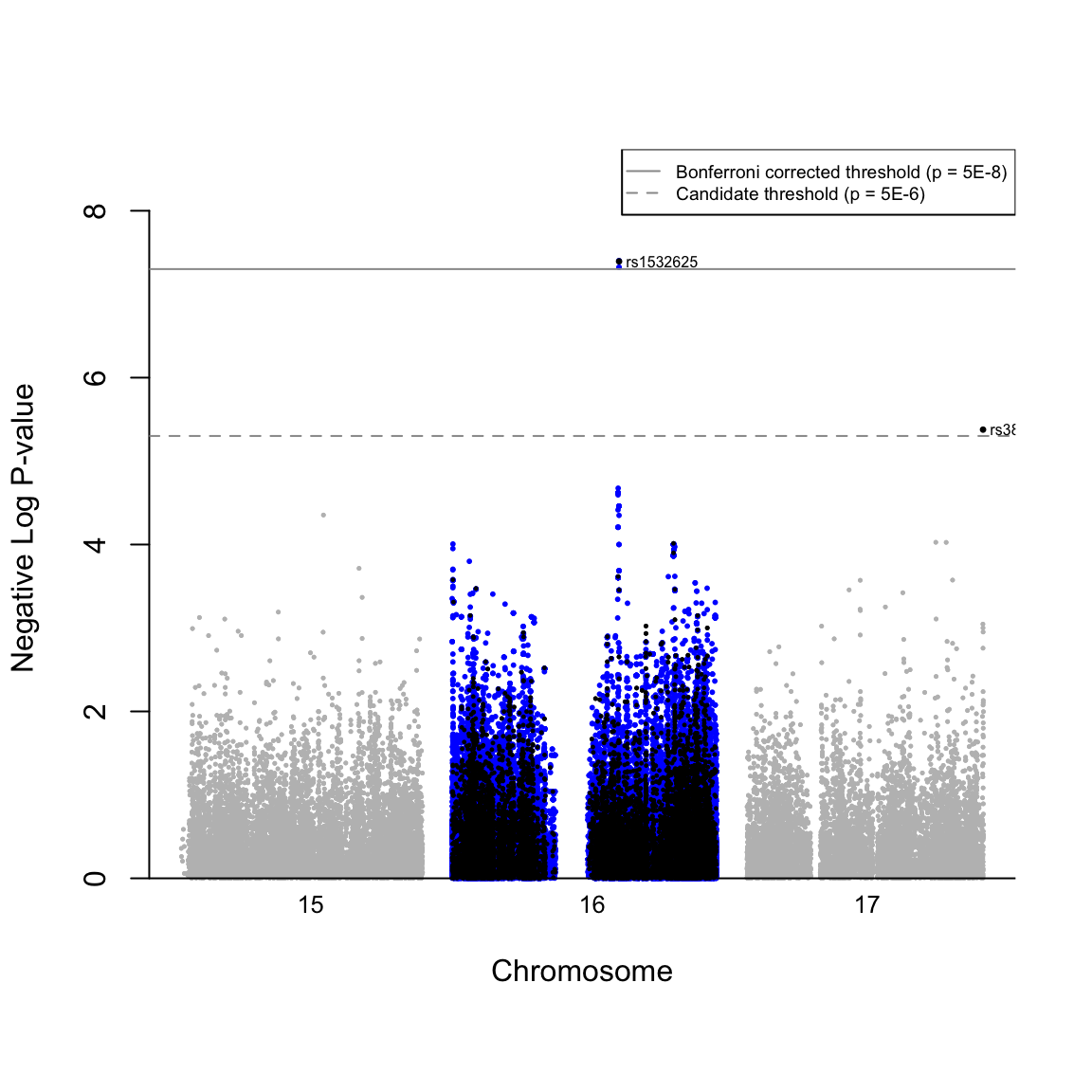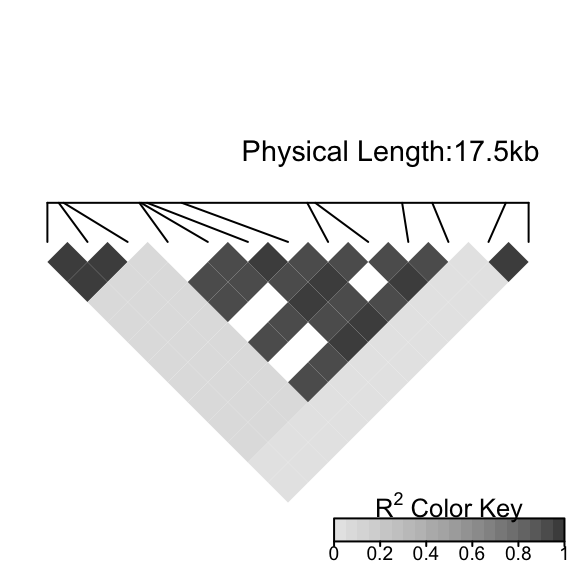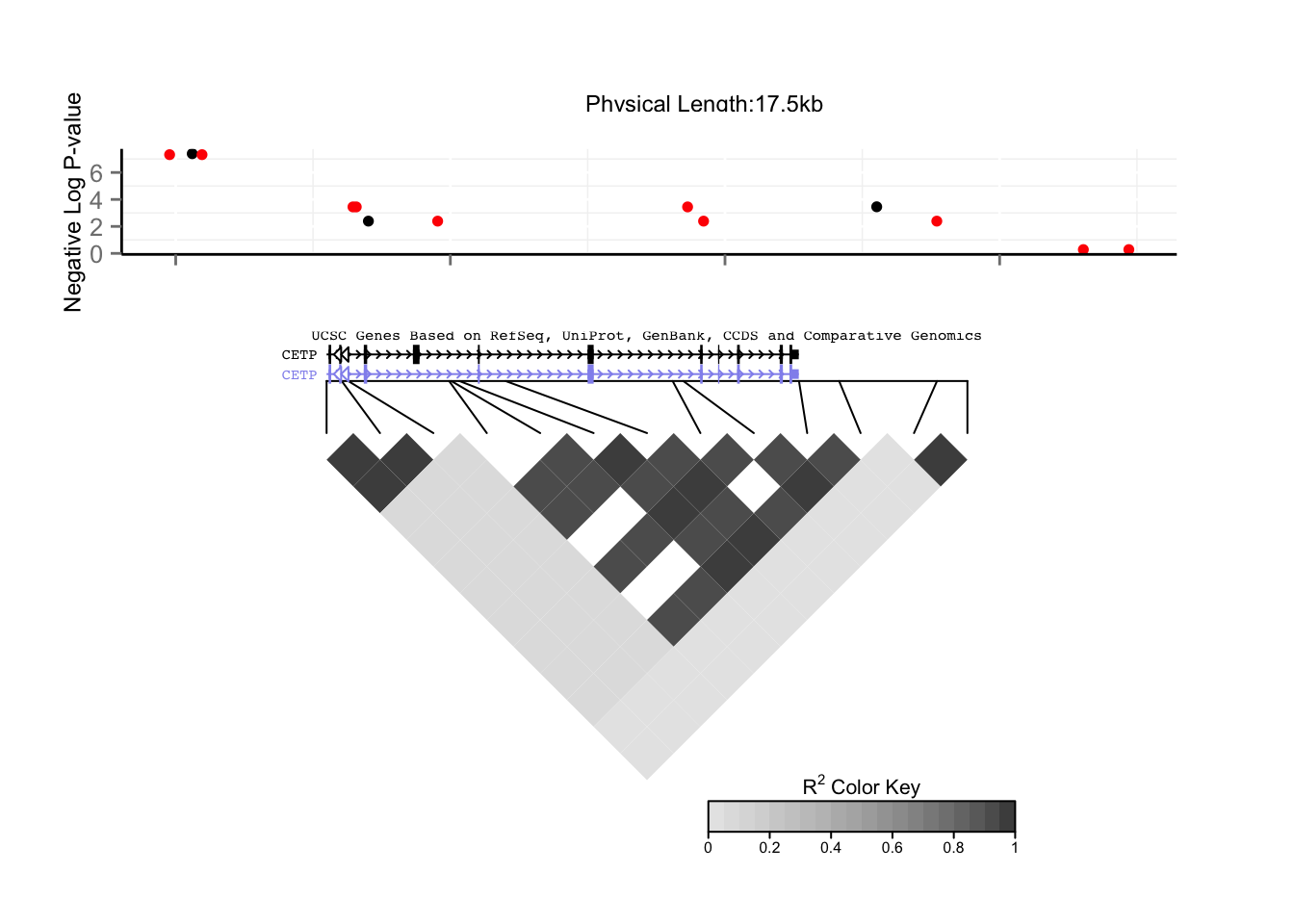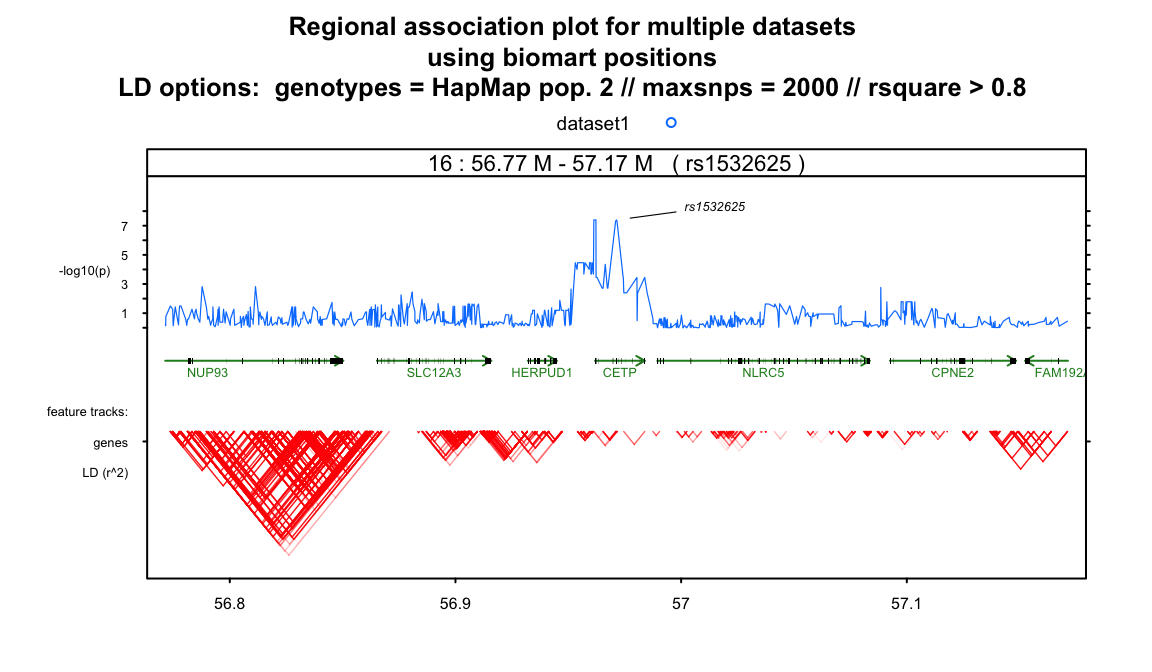## Post-analytic visualization

(This lab was adapted for statsTeachR by Sara Nunez, Nicholas Reich and Andrea Foulkes from our GWAS tutorial.)

There are several ways to visualize the results of a GWAA as well as gain more insight into the SNPs under study. Now that the analysis is complete and we have checked that our results are uninfluenced by confounding effects, we can start to look at our results more closely. Presented in this lab are three popular means of data visualization, commonly found in published GWAA papers. For convenience, we’ve made the code for these plots publically available, but chose not to present the details directly here. In the subsequent sections, we give details regarding the purpose and interpretations of manhattan plots, heatmaps and regional association plots. Note that we only ran analysis on chromosomes 15-17, and that in practice, these plots would display all 22 non-autosomal chromosomes.

### Getting started

library(downloader)
library(LDheatmap)
library(rtracklayer)
library(ggplot2)
library(snpStats)
library(plyr)
library(devtools)
install_url("http://cran.r-project.org/src/contrib/Archive/postgwas/postgwas_1.11.tar.gz")
library(postgwas)
load("m4_lab2_save.RData")

### Manhattan plots

Manhattan plots are used to visualize GWA significant results by chromosome location. It is a common way to take a glance at where signal is coming from and how strong it is. Often, significant results are highlighted in a different color (although here they are not), and a significance threshold line is presented. It is common practice to plot the negative log p values on the y-axis, with genomic location on the x-axis.

In this lab, we set up a plot for chromosomes 15-17, as these are the chromosomes we ran analysis on. Don’t forget we also have imputed data available for chromosome 16. This will be evident in the plot, as the function distinguishes between typed and imputed results through color code.

The first thing we want to do is download the function source code using the download() function from downloader. We then call the function to generate the plot. The function itself only takes the combined GWAS results as input.

code <- download("https://www.mtholyoke.edu/courses/afoulkes/Data/statsTeachR/GWAS_manhattan.R",
destfile="GWAS_manhattan.R")

source("GWAS_manhattan.R")

GWAS_Manhattan(GWAScomb)The first thing you probably notice in this plot are the different colors. The black and grey colors are used to distinguish between chromosomes. When all 22 chromosomes are plotted, you can imagine this becomes very helpful. There are also blue dots represented on chromosome 16. Recall that we only imputed SNPs on this chromosome, so these points represent the imputed SNP p values. There are also two threshold lines presented, one for the candidate cutoff and one for the bonferroni corrected cutoff. You’ll also notice that significant typed SNPs are labeled with their rs numbers.

#### Exercise

What does the gap on chromosome 16 represent? What possible reasons are there for it?

### Heatmaps

Heatmaps are typically used in the context of GWA analysis to visualize the linkage disequilibrium pattern between significant SNPs other SNPs in nearby regions. Here, for demonstrative purposes, we will look at the LD structure between our strongest SNP and the rest of the SNPs in the CETP gene.

The first thing we want to do is combine the genotypes for the typed and imputed SNPs in the CETP data frame. We then subset for SNPs that have appropriate genotypes 0, 1, 2 or NA. Recall that the target SNP matrix contains the genotypes for typed SNPs on chromosome 16, and the impCETPgeno contains the imputed genotypes in the CETP gene.

subgen <- cbind(target[,colnames(target) %in% CETP$SNP], impCETPgeno) # Subset SNPs for certain genotypes certain <- apply(as(subgen, 'numeric'), 2, function(x) { all(x %in% c(0,1,2,NA)) }) subgen <- subgen[,certain] #### Exercise How many SNPs were removed due to inadequate imputed genotypes? How many are left for the heatmap? Next, we subset the CETP data frame for the remaining SNPs, and order them by their position. This order is then used to reorder the columns of subgen. We do this because like the manhattan plot, the heatmap displays genomic location, so we need the SNPs to be in the order that they appear in the gene. CETP <- CETP[CETP$SNP %in% colnames(subgen),]

CETP <- arrange(CETP, position)

subgen <- subgen[ ,order(match(colnames(subgen),CETP$SNP))] Now that the data is in the correct order and we have only SNPs with appropriate genotypes, we can calculate the LD between them using an $$R^2$$ estimation. Once this is complete, we have enough data to display a simple heatmap. # Create LDheatmap ld <- ld(subgen, subgen, stats="R.squared") ll <- LDheatmap(ld, CETP$position, flip=TRUE, name="myLDgrob", title=NULL)The darker shaded regions in the above plot represent SNPs with higher LD (high $$R^2$$ values), while the lighter regions represent SNPs with low LD.

#### Exercise

What does a high $$R^2$$ value tell you about two SNPs?

#### Exercise

What relationship do you see between genetic distance and LD?

While this heatmap does tell us something about genetic distance and LD, it doesn’t give us very much information about the SNPs themselves. Recall, we wanted to investigate the LD between our top SNP and surrounding SNPs in the same gene. To do this, we add two additional layers to the plot to tell us more of a story.

The first thing we add is a representation of the CETP gene itself. In papers, this is useful for putting the LD plot into genomic context. We will do this using the LDheatmap.addGenes() function, which retreives the genes and their locations from the UCSC Genome Browser.

After this, to bring our analysis results into context, we add a plot of the $$-log10$$ p values above the CETP gene representaton. We do this through the use of the ggplot2 package, and we color code the p values based upon whether or not they were imputed or typed. Here, we specify imputed SNPs to be red and typed SNPs to be black.

llplusgenes <- LDheatmap.addGenes(ll, chr = "chr16", genome = "hg19", genesLocation = 0.01)
plot.new()
llQplot2<-LDheatmap.addGrob(llplusgenes, rectGrob(gp = gpar(col = "white")),height = .34)
pushViewport(viewport(x = 0.483, y= 0.76, width = .91 ,height = .4))

grid.draw(ggplotGrob({
qplot(position, Neg_logP, data = CETP, xlab="", ylab = "Negative Log P-value",
xlim = range(CETP$position), asp = 1/10, color = factor(type), colour=c("#000000", "#D55E00")) + theme(axis.text.x = element_blank(), axis.title.y = element_text(size = rel(0.75)), legend.position = "none", panel.background = element_blank(), axis.line = element_line(colour = "black")) + scale_color_manual(values = c("red", "black")) }))#### Exercise Why are there SNPs included in this heatmap that are outside of the CETP gene? #### Exercise Where is our top SNP (rs1532625) located? Is it in strong LD with other SNPs? If so, were they typed or imputed SNPs? ### Regional association plots Similar to the LD heatmap above, a regional association plot allows for visualization of SNP-wise signal accross a segment of a particular chromsome with the pairwise correlation between SNPs. In contrast to the heatmap, however, the regional assoication plots typically show a larger portion of the genome. Therefore, for plot legibility, LD calculations to be displayed can be selected based on pairwise SNP proximity and minimum LD. In this example we demonstrate a regional plot created by the regionalplot() function from postgwas. This function can use HapMap data downloaded from Ensembl, for LD calculations. By default it will use the most recent Genome Reference Consortium human genome build. Therefore, if we wish to use build GRCh37 (hg19), we will have to create a custom biomartConfigs object to retrieve the appropriate data. First, we must make a few edits to ensure that the plot works correctly. We start by making a data frame of the most significant SNP only. Then, we want to rename the columns of our compined output data frame for the plot function. Finally, we want to edit the biomartConfigs object as described above. snps<-data.frame(SNP=c("rs1532625")) GWAScomb <- rename(GWAScomb, c(p.value="P", chr="CHR", position="BP")) # Edit biomartConfigs so regionalplot function # pulls from human genome build 37/hg19 myconfig <- biomartConfigs$hsapiens
myconfig$hsapiens$gene$host <- "grch37.ensembl.org" myconfig$hsapiens$gene$mart <- "ENSEMBL_MART_ENSEMBL"
myconfig$hsapiens$snp$host <- "grch37.ensembl.org" myconfig$hsapiens$snp$mart <- "ENSEMBL_MART_SNP"

Now we can use the following code to create a regional association plot, which will display the same data as the LD plot, but on a larger scale and in a slightly different format.

regionalplot(snps, GWAScomb, biomart.config = myconfig,
window.size = 400000,
draw.snpname = data.frame(
snps = c("rs1532625"),
text = c("rs1532625"),
angle = c(20),
length = c(1),
cex = c(0.8)
),
ld.options = list(
gts.source = 2,
max.snps.per.window = 2000,
rsquare.min = 0.8,
show.rsquare.text = FALSE
),
out.format = list(file = NULL, panels.per.page = 1))#### Exercise

Describe the regional plot. What are some similarities and differences between this plot and the heatmap? Which do you prefer?